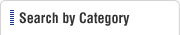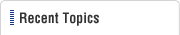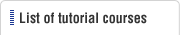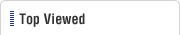HOME  > Low Cost Automation Tutorial  > #030 Regarding Moment of Inertia
Low Cost Automation Tutorial

#030 Regarding Moment of Inertia

Category : Drive mechanism design
February19, 2010

In motion mechanism designs, moment of inertia, angular acceleration, and friction torque may need to be evaluated in order to select actuator's outputs and sizes. Here, we shall get acquainted with some terms that appear in technical discussions of rotating machine elements such as ballscrews and cams.

(1) Moment of inertia

 Linear motion：F＝mxα　vs　Rotary motion：T＝Ixω ' 　　　F：Force 　　　m：Mass α：Acceleration 　　　T：Torque I：Moment of inertia 　　　ω ' ：Angular acceleration

It can be understood that Moment of Inertia (I) is an equivalent of the mass in linear motion.
This can be interpreted as a physical quantity of how difficult it would be to rotate an object or stop a rotating object. An object with large moment of inertia will require a motor with large stating torque as well as large rated torque in order to rotate.

(2) Another way to express the moment of inertia

The moment of inertia (I) is sometimes expressed in mass units（GD2）. In this case the moment of inertia (I) and GD2 can be expressed in a formula as below.

 GD2（kgfm2）＝4xgxI 　　　G：Object's mass（kg） 　　　D：Rotational diameter（m） 　　　g：Gravitational acceleration（m/s2）